Courses

# Test: Functions Of One,Two Or Three Real Variables - 4

## 20 Questions MCQ Test Topic-wise Tests & Solved Examples for IIT JAM Mathematics | Test: Functions Of One,Two Or Three Real Variables - 4

Description
This mock test of Test: Functions Of One,Two Or Three Real Variables - 4 for IIT JAM helps you for every IIT JAM entrance exam. This contains 20 Multiple Choice Questions for IIT JAM Test: Functions Of One,Two Or Three Real Variables - 4 (mcq) to study with solutions a complete question bank. The solved questions answers in this Test: Functions Of One,Two Or Three Real Variables - 4 quiz give you a good mix of easy questions and tough questions. IIT JAM students definitely take this Test: Functions Of One,Two Or Three Real Variables - 4 exercise for a better result in the exam. You can find other Test: Functions Of One,Two Or Three Real Variables - 4 extra questions, long questions & short questions for IIT JAM on EduRev as well by searching above.
QUESTION: 1

### Convert the set x in roster form if set x contains the positive prime number, which divides 72.

Solution:

2 and 3 are the divisors of 72, which are prime. So, the roster form of set x is (2, 3}.

QUESTION: 2

### Let f(x + y) = f(x)f(y) for all x and y and f(5) = - 2 and f'(0) = 3. What is the value of f'(5)?

Solution:

f'(0) = 3
implies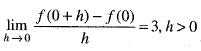or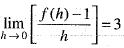[Since f(5 + 0) = f(5) • f(0)
implies f(5) = f(5) - f(0)
implies f(0) = 1]
Now, we have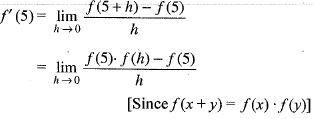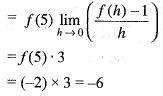[by eq. (1)]
Hence, f'(5) = -6

QUESTION: 3

### Let l  = { 1 } ∪ { 2 }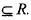For x ∈ R, let φ(x) = dis (x, l) = ln f{| x —y | : y ∈ l} then

Solution:

Given that,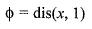= ln f{|x -.y| : y ∈ 1}
where l = { l } ∪ { 2 }
= ln f{| x -l| ,| x -2 | }
the graph of the φ(x) is given by.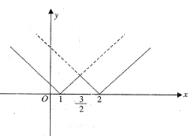Clearly, the graph of the function have sharp edges at x = 1,3/2 and 2. Therefore, f(x) is not differentiable at x
= 1, 3/2 and 2.
Hence, φ(x) is continuous on R but not
differentiable at x = 1, 3/2 and 2

QUESTION: 4

Let f(x) =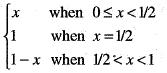then f is

Solution: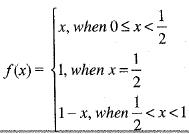At x =1/2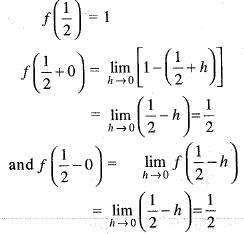Since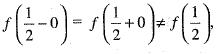therefore, f(x) is not continuous x =1/2

QUESTION: 5

If the function defined as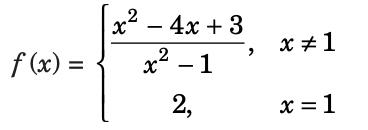Then, the function f (x) has

Solution: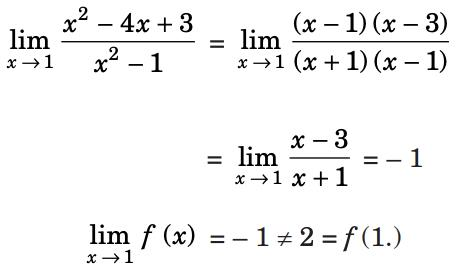So, the function is not continuous at the point x = 1. The discontinuity is of the first kind and can be removed by defining function as f (x) = – 1.

QUESTION: 6

Let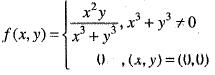then,

Solution:
QUESTION: 7

Let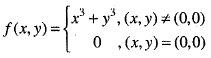then,

Solution:
QUESTION: 8

Let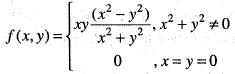then,

Solution:
QUESTION: 9

Let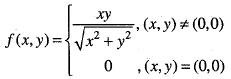then,

Solution:
QUESTION: 10

Let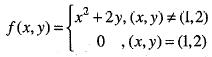then,

Solution:
QUESTION: 11

Consider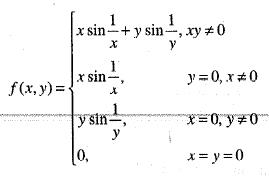then,

Solution:
QUESTION: 12

If we expand sinx by Taylor’s series about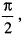then a1, a7, a4, a3 are

Solution:

Given function f(x) = sin x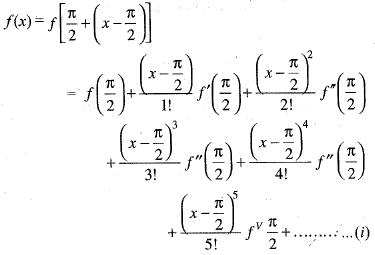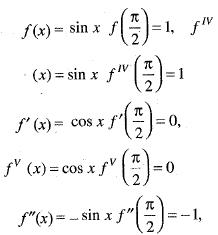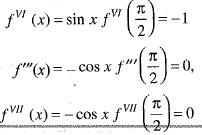the value of a2, a7 a4, a3 in (i) are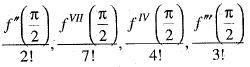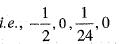QUESTION: 13

If f(x) is twice differentiable and | f(x) | < α, f'(x)| < β, in the range x > α, then which of the following is correct?

Solution:

Let x > a and n a positive number then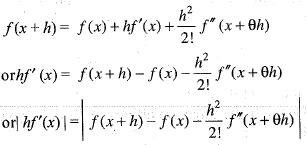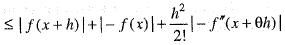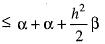or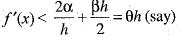Now, for maxima or minima of f(h), we have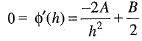or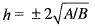and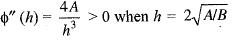Hence, the least value of φ(h)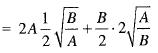= 2√AB
thus,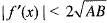QUESTION: 14

The function f(x) = | x + 2 | is not differentiable at a point

Solution:

Given that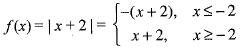Clearly, the rule of the function is changing at x = 0, so we shall test the differentiability of f(x) only at the point x = -2 obviously, being polynomial, at all other points the function is differentiable.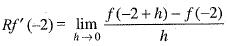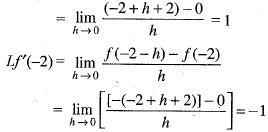Since Lf '(- 2) ≠ R'f(- 2) , therefore , f(x) is not differentiable at x = - 2 .

QUESTION: 15

Using Rolle’s theorem, the equation.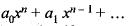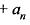= 0 has atleast one root between 0 and 1, if

Solution:

Consider the function f defined by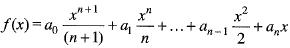Since , f(x) is a polynomial, it is continuous and differentiable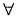x. Consequently f(x) is continuous in the closed interval [0, 1] and differentiable in the open interval [0,1] Also , f(0) = 0 and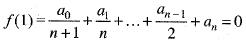i.e. , f(0) = f(1)
Thus, all the three conditions of Rolle’s theorem are satisfied
Hence, there is atleast one value of x in the open interval [0, 1]
where f'(x) = 0
i.e., cos xn + a1.xn-1 + . . . + an-1 + a= 0

QUESTION: 16

A function f : R-->R satisfies the e q .f(x +y) = f(x).f(y),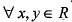and f(x) ≠ 0,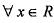. If f(x) is differentiate at 0 a n d f'(0 ) = 2 then f'(x) is equal to

Solution:

We have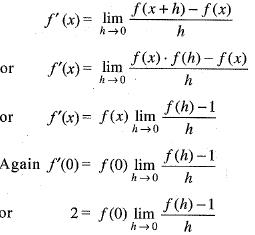Now f(0 + 0)= f(0).f(0)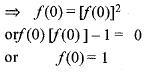[Since f(x)  0 ,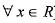]
from eq. (ii) we get
Gives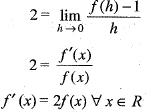QUESTION: 17

Which one of the following is true?

Solution:
QUESTION: 18

Let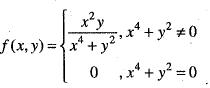then,

Solution:
QUESTION: 19

Let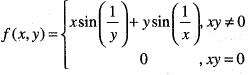then,

Solution:
QUESTION: 20

Let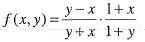then,

Solution:

Track your progress, build streaks, highlight & save important lessons and more!

### Similar Content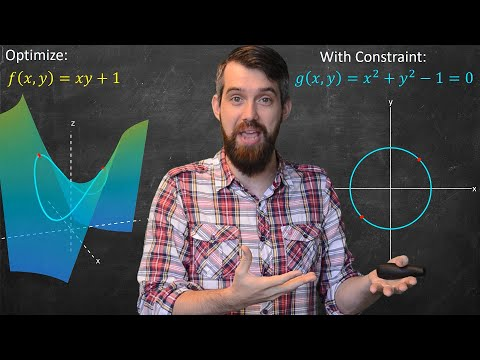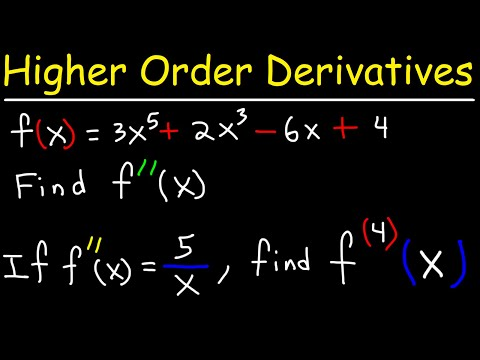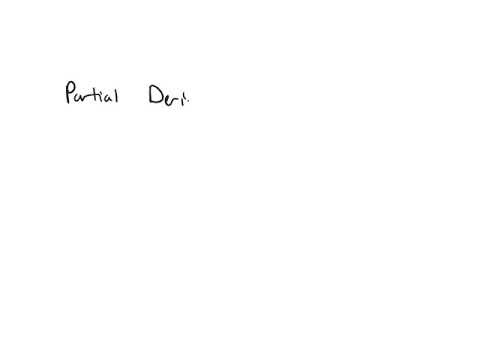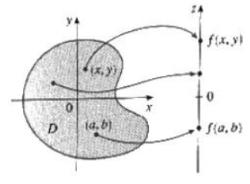### Related tests